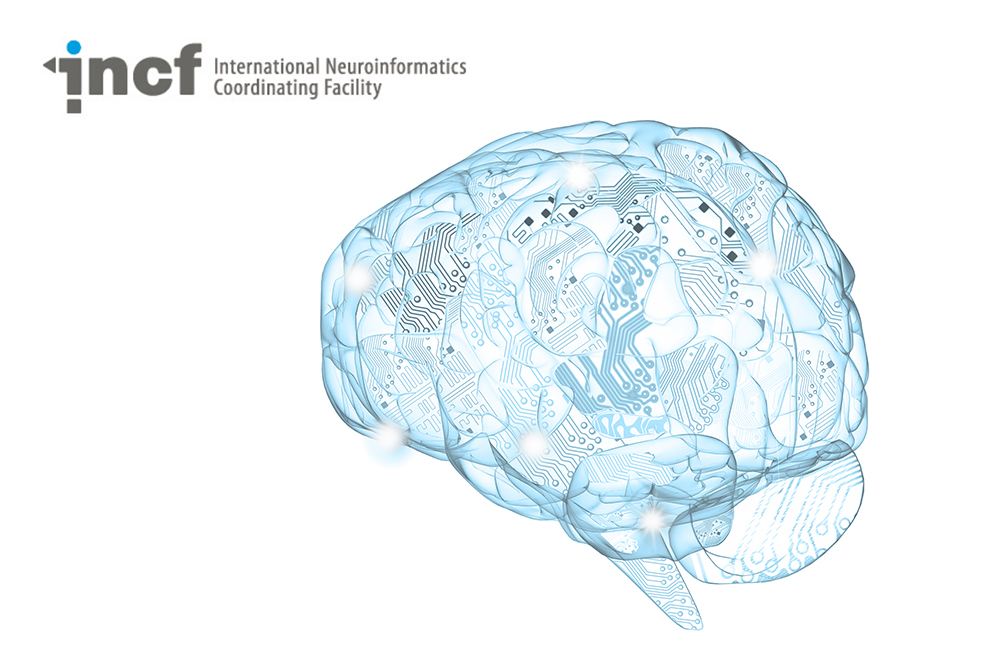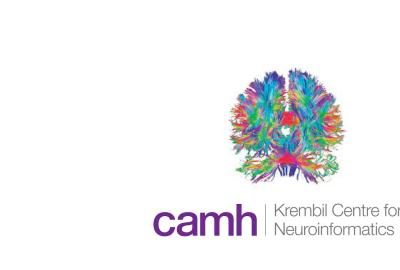# Introduction to Computational Neuroscience

By
Category
Level
Beginner

Most who enter the field of computational neuroscience have a prior background in either mathematics, physics, computer science, or (neuro)biology. Since computational neuroscience requires a bit of knowledge from all these fields, with some basic knowledge of neurons and a familiarity with certain types of equations and mathematical concepts, we recommend two different "starting tracks" depending on the student's background before you begin the lectures listed below:

Intro to computational neuroscience for a computer sci/math background
The student should learn basic concepts and equations for how neurons generate signals, either a more through introduction via the Cellular Mechanisms of Brain Function course or a quick reminder via the Basic Mathematics for Computational Neuroscience tutorials.

Intro to computational neuroscience for a biology background
Here the student is assumed to already have basic knowledge of neurons. We recommend some orientation in mathematics (differential equations, linear algebra, dynamical systems) and computer science. There are a number of possible online courses openly available, for instance the MIT OpenCourseware course on Differential Equations. After that, we recommend a quick orientation on how these mathematics apply to neuroscience by viewing the Basic Mathematics for Computational Neuroscience tutorials.

Course Features
Videos
Lectures
Tutorials
Slides
Lessons of this Course
1
1
Duration:
1:23:01

This lecture provides an introduction to neuron anatomy and signaling, and different types of models, including the Hodgkin-Huxley model.

2
2
Duration:
8:23

This lecture describes non-spiking simple neuron models used in artificial neural networks and machine learning.

3
3
Duration:
48 Slides
Speaker:

This lesson provides an introduction to simple spiking neuron models.

4
4
Duration:
28:29

In this lesson you will learn about the ionic basis of the action potential, including the Hodgkin-Huxley model.

5
4
Duration:
1:11:29
Speaker:

This lesson provides an overview of plasticity on many levels, including short-term, long-term, metaplasticity, and structural plasticity. The lesson also provides xamples related to modelling of biochemical networks.

Note: The sound uptake is a bit noisy the first few minutes, but gets better from about 5 mins in

6
6
Duration:
1:00:11
Speaker:

This lesson gives an introduction to the modelling of chemical computation in the brain.

7
7
Duration:
15:44

In this lesson you will hear about several computationally demanding studies of synaptic plasticity on the molecular level.

8
8
Duration:
1:26:06

This lesson gives an introduction to stability analysis of neural models.

9
9
Duration:
1:25:38

This lesson continues from the previous lectures, providing introduction to stability analysis of neural models.

10
10
Duration:
1:24:30

In this lesson, you will learn about phenomena of neural populations such as synchrony, oscillations, and bursting.

11
11
Duration:
1:31:57

This lesson continues from the previous lecture, giving an overview of various neural phenomena such as oscillations and bursting.

12
12
Duration:
1:26:02

This lesson provides more context around weakly coupled oscillators.

13
13
Duration:
1:24:44

This lesson builds upon previous lectures in this series, providing an overview of coupled oscillators.

14
14
Duration:
1:26:42

In this lesson, you will learn about neuronal models based on their spike rate.

15
15
Duration:
1:20:42

In this lesson, you will learn about neural activity pattern generation in visual system hallucinations.

16
16
Duration:
19:26
Speaker:

This lesson provides an introduction to the role of models in theoretical neuroscience.

17
17
Duration:
39:09
Speaker:

This lesson introduces different types of models, model complexity, and how to choose an appropriate model.

18
18
Duration:
1:22:11

This lesson gives an overview of balanced excitatory-inhibitory (E-I) networks, stability, and gain modulation.

19
19
Duration:
1:16:47
Speaker:

In this lesson, you will learn about methods for dimensionality reduction of data, with a focus on factor analysis.

20
20
Duration:
1:39:32

This lesson gives an in-depth look into various types of neuronal networks, as well properties, parameters, and phenomena which characterize them.

21
21
Duration:
1:24:22

In this lesson, you will learn about spiking neuron networks and linear response models.

22
22
Duration:
1:12:38
Speaker:

This lesson discusses Bayesian neuron models and parameter estimation.

23
23
Duration:
1:33:34

This lesson gives an overview of Bayesian memory and learning, as well as how to go from observations to latent variables.

24
24
Duration:
1:34:42

In this lesson, you will learn about how constraints can help us understand how the brain works.

25
25
Duration:
1:29:38

This lesson discusses how to approach neural systems from an evolutionary perspective.

##### Recent courses#### Reproducible Science (Including Git, Docker, and Binder)#### Simulating Brain Microcircuit Activity and Signals in Mental Health